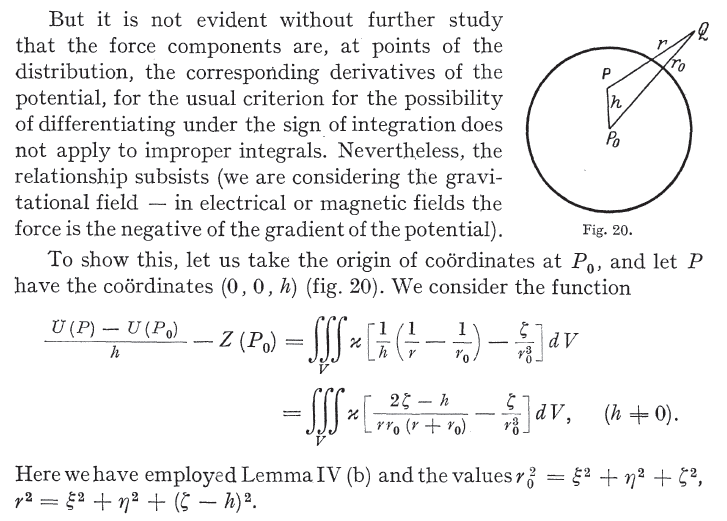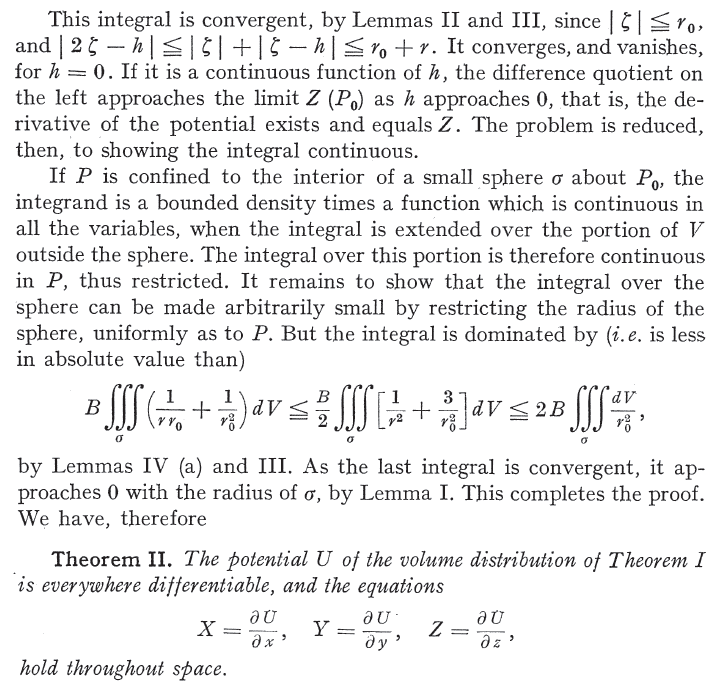# Is Leibniz integral rule allowed in this potential improper integral?

• I
Electric potential at a point inside the charge distribution is:

##\displaystyle \psi (\mathbf{r})=\lim\limits_{\delta \to 0} \int_{V'-\delta}

\dfrac{\rho (\mathbf{r'})}{|\mathbf{r}-\mathbf{r'}|} dV'##

where:

##\delta## is a small volume around point ##\mathbf{r}=\mathbf{r'}##
##\mathbf{r}## is coordinates of field point
##\mathbf{r'}## is coordinates of source point
##\rho (\mathbf{r'})## is the density of charge distribution

##\displaystyle \nabla \psi (\mathbf{r}) =\nabla\ \left[ \lim\limits_{\delta \to 0} \int_{V'-\delta} \dfrac{\rho (\mathbf{r'})}{|\mathbf{r}-\mathbf{r'}|} dV' \right] =\lim\limits_{\delta \to 0} \int_{V'-\delta} \rho (\mathbf{r'})\ \nabla \left( \dfrac{1}{|\mathbf{r}-\mathbf{r'}|} \right) dV'##

In the last step, we have applied Leibniz integral rule (basic form).

The validity of this technique for improper integrals is discussed below:

The following passage from the book "Foundations of Potential Theory page 151" says the technique is not valid. But it says the equation ##\mathbf{E}=-\nabla \psi## still holds at points inside source regions ##V'##. It also gives a "little" proof of the argument.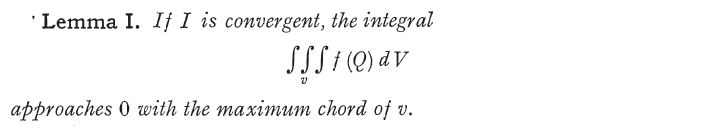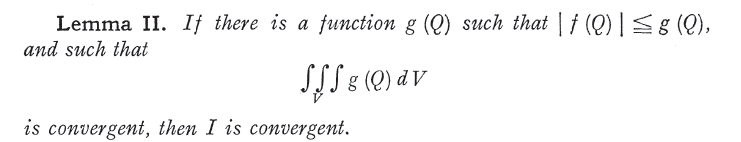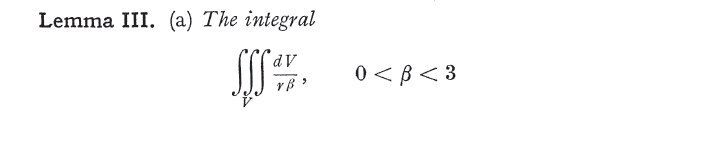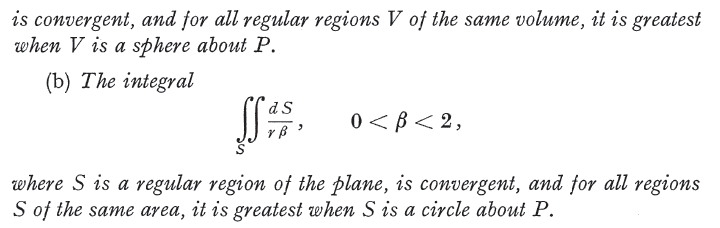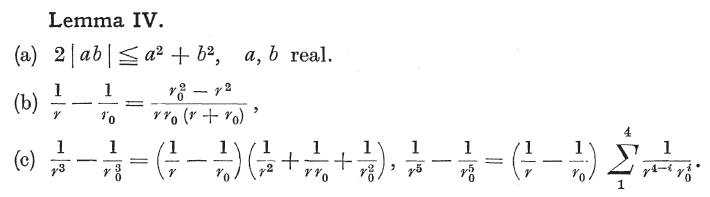(continued below)